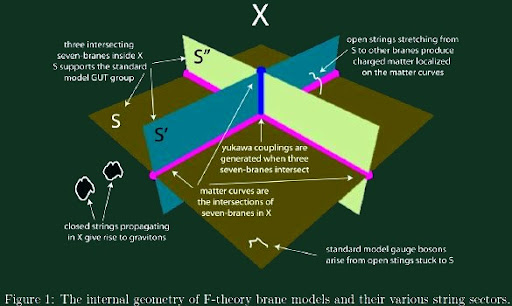## Tuesday, June 24, 2014 ...//### An LHC-friendly type IIA stringy braneworld

Dimitri Nanopoulos et al. have studied a different class of string compactifications capable of describing the Universe around us, at least as the first sketch. But today, they switched to type IIA braneworlds:

A Realistic Intersecting D6-Brane Model after the First LHC Run
Tianjun Li, D. V. Nanopoulos, Shabbar Raza, Xiao-Chuan Wang look at a particular model with D6-branes in type IIA string theory on the $$T^6/ \ZZ_2\times\ZZ_2$$ orbifold.This picture is originally from a paper about F-theory model building but the 2D illustrations aren't too different.

And this model ends up being compatible with the observations at the LHC so far.

I never quite understand such semi-stringy, semi-phenomenological papers. They seem to talk about one particular string model but they also scan for "parameter spaces". A particular string theory vacuum doesn't produce any parameter spaces. It is 100 percent predictive – string theory is the ultimate predictive theory one may envision – so it fixes all the parameters.

At any rate, they must perform some "incomplete extraction" of the terms and parameters from the string model so that some effective theory with unknown parameters is left. Assuming the Higgs boson in the $$123-127\GeV$$ range, the predicted superpartner masses are:
• top squark: $$0.5-1.2\TeV$$
• stau: $$0.8\TeV$$
• gluino: $$3-18\TeV$$
• squarks$${}_{1G,2G}$$: $$3-14\TeV$$
• sleptons$${}_{1G,2G}$$: $$2-7\TeV$$
You may see that some particles may be accessible to the LHC, others might want to wait for a bigger machine.

Incidentally, a different paper tries to explain the DAMA signal using some Dirac dark matter. There's also a paper about a generalized axion possibly explaining the $$3.55\keV$$ line and another paper constructing a radiative neutrino model for the same line.

ATLAS claims to have excluded top squarks in $$173-177\GeV$$.

In the theoretical arXiv, Balasubramanian et al. coin "entwinement", some weird cousin or version of entanglement possibly relevant for the emergence of spacetimes in quantum gravity, a concept that I haven't understood yet.

You may recheck the renewed LHC restart schedule at the bottom of that page.#### snail feedback (0) :

(function(i,s,o,g,r,a,m){i['GoogleAnalyticsObject']=r;i[r]=i[r]||function(){ (i[r].q=i[r].q||[]).push(arguments)},i[r].l=1*new Date();a=s.createElement(o), m=s.getElementsByTagName(o);a.async=1;a.src=g;m.parentNode.insertBefore(a,m) })(window,document,'script','//www.google-analytics.com/analytics.js','ga'); ga('create', 'UA-1828728-1', 'auto'); ga('send', 'pageview');